# A Mathematical Model for Non-Linear Structural Analysis Reinforced Beams of Composite Materials

A Mathematical Model for Non-Linear Structural Analysis Reinforced Beams of Composite Materials
Mechanical Technology Department, Mosul Technical Institute, Northern Technical University, Mosul 41000, Iraq

Power Mechanical Techn. Eng. Dept., Tech. Engineering College-Northern Technical University, Mosul 41000, Iraq

Corresponding Author Email:
Page:
311-333
|
DOI:
https://doi.org/10.18280/mmep.100137
6 October 2022
|
Accepted:
25 December 2022
|
Published:
28 February 2023
| Citation

OPEN ACCESS

Abstract:

The beams are frequently utilized in construction as well as in the fabrication of vehicles like as trains, ships, and airplanes. Depending on the necessary working circumstances, several materials may have been utilized in the production of these beams, from high fatigue resistance, high corrosion resistance, strong earthquake resistance, and other aspects. As a result, composite beams made of glass or carbon fibers are increasingly commonly employed. This is a result of its strong collapse resistance, light weight, and strong fatigue stress resistance. In order to compare the models' resistance to deformations, stresses, and strains that they are exposed to during loading, this article focuses on constructing a variety of models using a variety of composite materials and shapes. The outcomes demonstrate a rise in the rate of deformation. against beams with linear shapes in those with non-linear shapes. Additionally, the findings demonstrate an increase in stresses and strains in regions with curves (i.e., areas that are nonlinear).

Keywords:

fiberglass, carbon fiber, steel, concrete, beams, Finite element method

1. Introduction

In this article, nine mathematical models of beams will be designed and made up of different composite materials and in different shapes (straight, twisted, arched), in order to compare between deformations, stresses and strains when a high load is placed on them using the ANSYS-15.0 program, and this study differs from previous studies because it takes Linear and other non-linear models have the same dimensions, and the results are compared for all models. The last paragraphs of the article are as follows: The material used and the mathematical model, Results and Discussion, Conclusions, Acknowledgment, future studies and references.

2. Materials and Model Analysis

The ANSYS-15 program was used to create nine 3D models of three different types of beams. Three models are made of traditional materials (Concrete and Rebar), and the three models are made of composite materials (T300 Carbon Fiber and 7901 Epoxy Resin), and three models are composed of composite materials (E-Glass Fibre and 7901 Epoxy Resin), and exerting a force load (10 MN) in the direction of the vertical axis (y) into the center of the top surface of each of the nine models.

## 1.png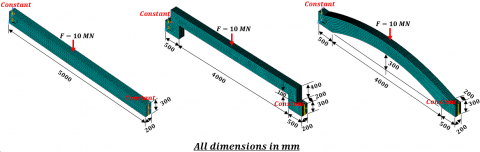Figure 1. It shows the shapes of the models used in the tests and the points of loading them

Table 1. Describe the mechanical properties of fiberglass, jute, carbon fiber, and concrete composition [18, 29-33]

## 53.png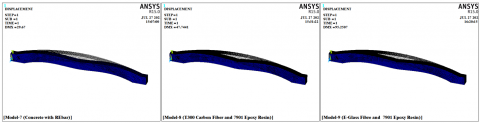Figure 53. Results of the deflection (δ), for models (7, 8, and 9)

## 54.png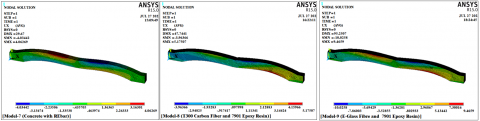Figure 54. Results of the displacement in a direction component (Ux), for models (7, 8, and 9)

## 55.png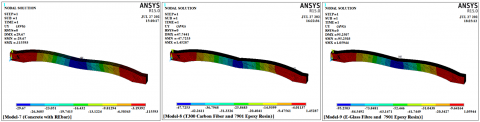Figure 55. Results of the displacement in a direction component (Uy), for models (7, 8, and 9)

## 56.png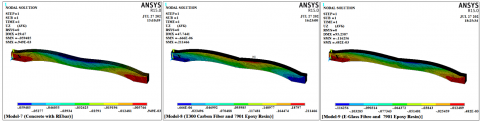Figure 56. Results of the displacement in a direction component (Uz), for models (7, 8, and 9)

## 57.pngFigure 57. The results of normal stress (σx), for models (7, 8, and 9)

## 58.png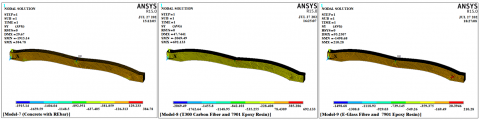Figure 58. The results of normal stress (σy), for models (7, 8, and 9)

## 59.png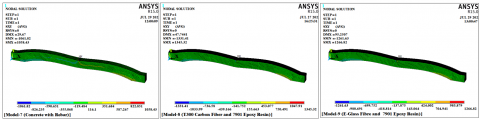Figure 59. The results of shear stress (τxy), for models (7, 8, and 9)

## 60.png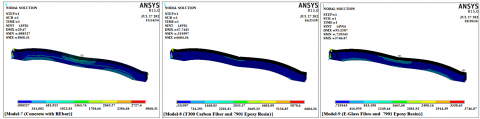Figure 60. The results of intensity stress (σint.), for models (7, 8, and 9)

## 61.png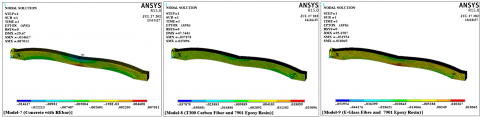Figure 61. The results of normal strain (εx), for models (7, 8, and 9)

## 62.png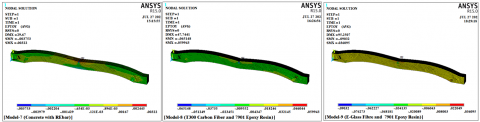Figure 62. The results of normal strain (εy), for models (7, 8, and 9)

## 63.png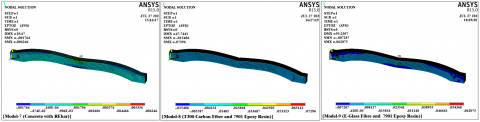Figure 63. The results of normal strain (εz), for models (7, 8, and 9)

## 64.png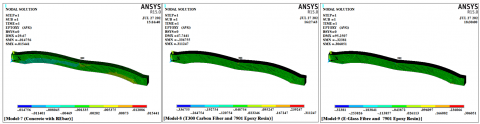Figure 64. The results of shear strain (εxy), for models (7, 8, and 9)

## 65.png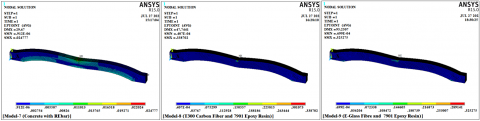Figure 65. The results of intensity strain (εint.), for models (7, 8, and 9)

## 66.png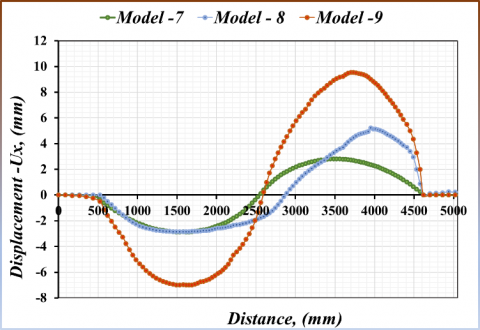Figure 66. Compare the results of the relationship between displacement (Ux) and distance on a nonlinear path (C-C), for models (7, 8, and 9)

## 67.png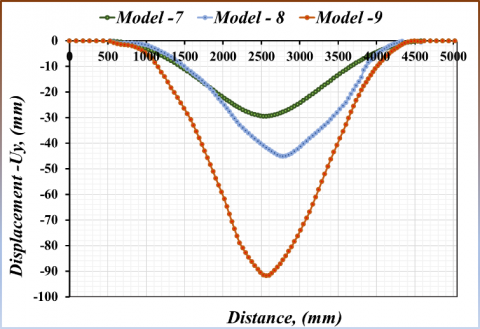Figure 67. Compare the results of the relationship between displacement (Uy) and distance on a nonlinear path (C-C), for models (7, 8, and 9)

## 68.png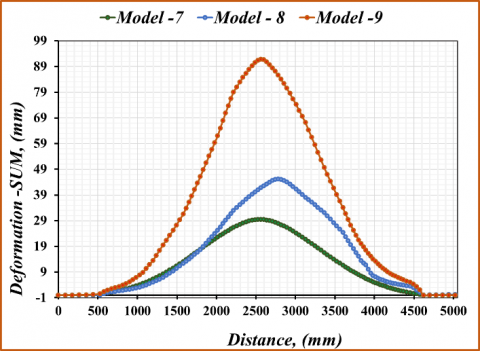Figure 68. Compare the results of the relationship between displacement (Usum) and distance on a nonlinear path (C-C), for models (7, 8, and 9)

## 69.png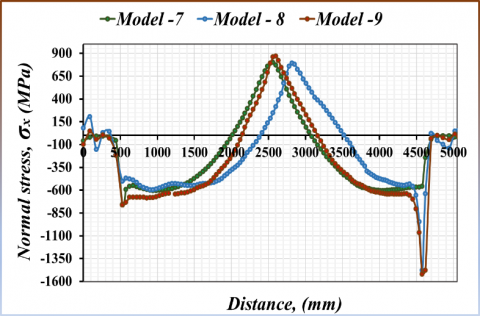Figure 69. Compare the results of the relationship between normal stress (σx) and distance on a nonlinear path (C-C), for models (7, 8, and 9)

## 70.png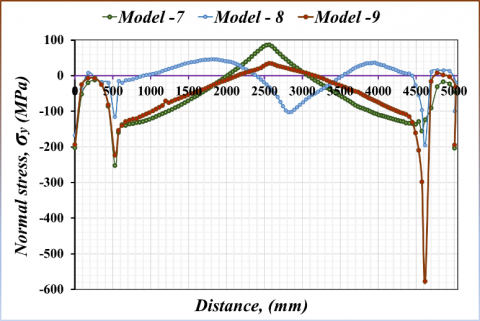Figure 70. Compare the results of the relationship between normal stress (σy) and distance on a nonlinear path (C-C), for models (7, 8, and 9)

## 71.png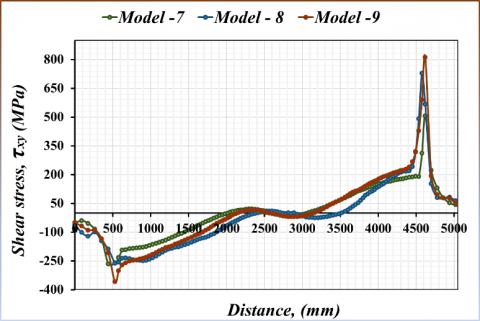Figure 71. Compare the results of the relationship between shear stress (τxy) and distance on a nonlinear path (C-C), for models (7, 8, and 9)

## 72.pngFigure 72. Compare the results of the relationship between stress intensity (σint.) and distance on a nonlinear path (C-C), for models (7, 8, and 9)

## 73.png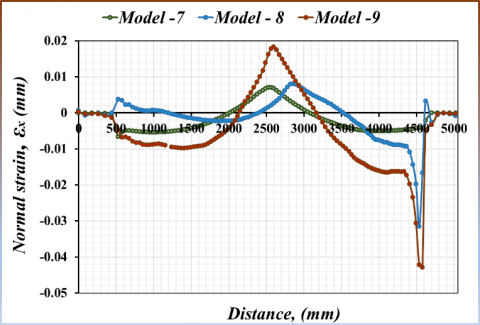Figure 73. Compare the results of the relationship between normal strain (εx) and distance on a nonlinear path (C-C), for models (7, 8, and 9)

## 74.png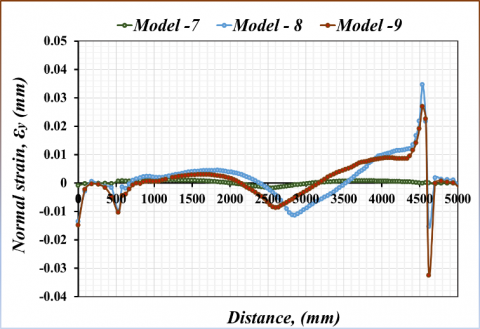Figure 74. Compare the results of the relationship between normal strain (εy) and distance on a nonlinear path (C-C), for models (7, 8, and 9)

## 75.png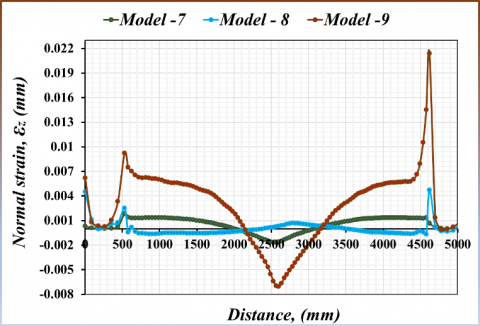Figure 75. Compare the results of the relationship between normal strain (εz) and distance on a nonlinear path (C-C), for models (7, 8, and 9)

## 76.png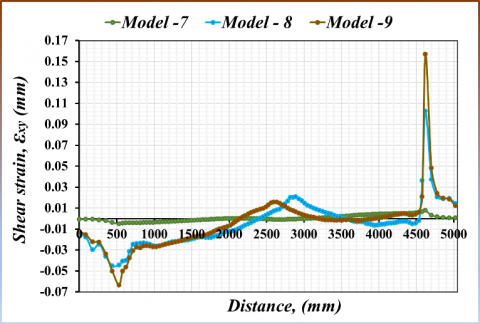Figure 76. Compare the results of the relationship between shear strain (εxy) and distance on a nonlinear path (C-C), for models (7, 8, and 9)

## 77.png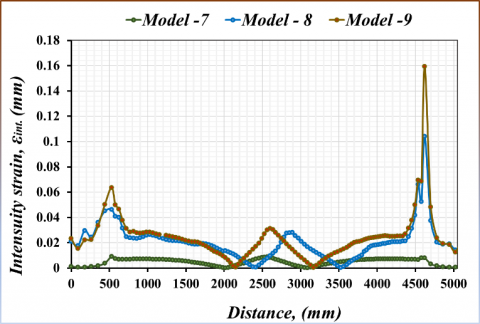Figure 77. Compare the results of the relationship between intensity strain (εint.) and distance on a nonlinear path (C-C), for models (7, 8, and 9)

4. Conclusions

The research of several composite materials that are shaped like beams of various shapes is presented in this work. by testing the strains, stresses, and deformations that these various beams experience when subjected to a certain load. The study also focused on the comparison between the results obtained, and the following was concluded from the analysis of the results:

1. The data analysis findings demonstrate that the model (M-6) had the largest deflection value, and its value (381.75 mm), while the model (M-7) and its value (29.67 mm) had the least deflection. The cause of this is that ductile failures occur far less frequently in pressure-controlled columns and beams. As an illustration, failure of the crushing kind in a shaft typically happens very quickly and without prior notice. Collars are used to increase safety since they restrict the movement of the crushed aggregate.

2. According to the study of the data, the nonlinear models (M-4, M-5, M-6, M-7, M-8, and M-9), have higher normal stresses (σx, σy, and σz) in the locations where there are curves, they have significant stress levels, but the linear models (M-1, M-2, M-3) such stresses don't exist. This is caused by the buildup of stresses in the regions where there are bends.

3. It is evident from the analysis of the findings that there are hardly any shear stresses (τxz, and τyz) in any of the models. While there are significant shear stresses (τxy) of this type in the non-linear models (M-4, M-5, M-6, M-7, M-8, and M-9), these stresses are concentrated in the model's curved regions. Whereas in the eighth model, the maximum value of negative shear stress values at point (786.66 MPa, 776.92 mm), while the ninth model's point (811.09 MPa, 4620.3) had the highest positive shear stress. This is because shear stresses develop where there are bends, but not in straight models, which are not exposed to shear stresses from vertical loading.

4. A thorough analysis of the data reveals that stress intensity (σint.) exists in several locations across the models, including their beginnings, middles, and ends as well as in their curved portions. In the second model, the two points ((3,950 MPa, 0 mm) & (3,950 MPa, 5,000 mm)) had the highest values of stress intensity (σint.). The reason for this is that the stress concentration increases in the curved areas, and when designing, this must be taken into account, and the design depends on the stresses in the curved areas.

5. According to the data, the normal strains (εx, εy and εz) in the linear models (1, 2, and 3) have high values at the start, middle, and end of the models, while the normal strains (εx, εy and εz) are distributed differently in nonlinear models (M-4, M-5, M-6, M-7, M-8, and M-9), particularly in curvilinear regions where they have significant strain values. This shows that it is possible to reduce the strains at the beginning, middle and end of the straight models, by changing them with other curved models to reduce the strains in the middle, beginning and end of the models to other controlled areas during the design.

6. The findings demonstrate that shear stresses (εxy) in linear models (1, 2, and 3) occur at the start and end of the models, whereas shear strains in non-linear models (M-4, M-5, M-6, M-7, M-8, and M-9) in places where there are curves. This shows that the shear strains are concentrated in the areas of curvature and other curved areas in the curved and non-rectilinear models as opposed to the straight ones.

7. The findings indicate that the beginning, middle, and ends of the linear models (1, 2, and 3) exhibit intensity strains (εint.), but the non-linear models (M-4, M-5, M-6, M-7, M-8, and M-9) exhibit intensity strains in the areas around the curves and middle models. This indicates that the strain concentrations in the curved and non-rectangular models occur in regions different from the strains that occur in the straight models.

5. Future Studies

In future studies, we suggest using different forces such as (20 MN, 30 MN, ........) to simulate different working conditions and reveal the effect of forces on the models, as well as another study using different sizes and the effect of the size of the model on the resistance of different materials, as well as experimental models can be built, tested and compared with the results obtained.

Acknowledgment

The Ministry of Higher Education and Scientific Research of the Republic of Iraq funded the Engineering Science Research Program through the Northern Technical University/Technical Institute of Mosul, which supported this study. (No. 00333- 2022).

References

 Shalall, M.A. (2017). Nonlinear analysis of continuous composite beam by finite element method. Journal of Engineering and Development, 9(2): 54-69.

 Yu, W.B. (2002). Variational asymptotic modeling of composite dimensionally reducible structures. Ph.D. Thesis, Aerospace Engineering, Georgia Institute of Technology: 1-8.

 Pai, P.J., Nayfeh, A.H. (1992). A nonlinear composite beam theory. Nonlinear Dynamics Journal, 3(4): 273-303. https://doi.org/10.1007/BF00045486

 Yu, W.B., Blair, M. (2012). GEBT: A general-purpose nonlinear analysis tool for composite beams. Composite Structures Journal, 94(9): 2677-2689. https://doi.org/10.1016/j.compstruct.2012.04.007

 Weinberg, M. (1987). Shear testing of neat thermoplastic resins and their unidi 315 rectional figureite composites. Composites, 18(5): 386-392. https://doi.org/10.1016/0010-4361(87)90363-6

 Jiang, F., Deo, A., Yu, W.B. (2018). A composite beam theory for modeling nonlinear shear behavior. Engineering Structures Journal, 155: 73-90. https://doi.org/10.1016/j.engstruct.2017.10.051

 Karakuzu, R., Icten, B.M., Tekinsen, O. (2010). Failure behavior of composite laminates with multi-pin loaded holes. Journal of Reinforced Plastics and Composites, 29(2): 247-253. https://doi.org/10.1177/0731684408097758

 Chang, F.K., Chang, K.Y. (1987). A progressive damage model for laminated composites containing stress concentrations. Journal of Composite Materials, 21(9): 834-855. https://doi.org/10.1177/002199838702100904

 Jiang, F., Yu, W. (2015). Nonlinear variational asymptotic sectional analysis of hyperelastic beams. AIAA Journal, 54(2): 679-690. https://doi.org/10.2514/1.J054334

 Lee, C.S., Lee, J.M. (2015). A study on the evaluation of fiber and matrix failures for laminated composites using hashin·puck failure criteria. Journal of the Society of Naval Architects of Korea, 52(2): 143-152. https://doi.org/10.3744/SNAK.2015.52.2.143

 Donadon, M.V., Iannucci, L., Falzon, B.G., Hodgkinson, J.M., de Almeida, S.F.M. (2008). A progressive failure model for composite laminates subjected to low velocity impact damage. Computers & Structures, 86(11-12): 1232-1252. https://doi.org/10.1016/j.compstruc.2007.11.004

 Lomakin, E.V., Fedulov, B.N. (2015). Nonlinear anisotropic elasticity for laminate composites. Meccanica, 50(6): 1527-1535. https://doi.org/10.1007/s11012-015-0104-5

 Žarković, D. (2022). Matrix 3d software for linear and non-linear structural analysis and design. Materials: Conference Contemporary Civil Engineering Practice, Serbia. https://www.researchgate.net/publication/361307277

 Grassl, P., Xenos, D., Nyström, U., Rempling, R., Gylltoft, K. (2013). CDPM2: A damage-plasticity approach to modelling the failure of concrete. International Journal of Solids and Structures, 50(24): 3805-3816. https://doi.org/10.1016/j.ijsolstr.2013.07.008

 Karash, E.T., Sultan, J.N., Najem, M.K. (2021). The difference in the wall thickness of the helicopter structure are made of composite materials with another made of steel. Mathematical Modelling of Engineering Problems 9(2): 313-324. https://doi.org/10.18280/mmep.090204

 Najim, M., Sultan, J., Karash, E. (2020). Comparison of the resistance of solid shell of composite materials with other solid metal Materials. In: IMDC-SDSP 2020, pp. 28-30. https://doi.org/10.4108/eai.28-6-2020.2298518

 Žarković, D., Jovanović, Đ., Vukobratović, V., Brujić, Z. (2019). Convergence improvement in computation of strain-softening solids by the arc-length method. Finite Elements in Analysis and Design, 164: 55-68. https://doi.org/10.1016/j.finel.2019.06.005

 Karash, E.T., Alsttar Sediqer, T.A., Elias Kassim, M.T. (2021). A comparison between a solid block made of concrete and others made of different composite materials. Revue des Composites et des Matériaux Avancés, 31(6): 341-347. https://doi.org/10.18280/RCMA.310605

 Karash, E.T. (2011). Modelling of unilateral contact of metal and fiberglass shells. Applied Mechanics and Materials Journal, 87: 206-208. https://doi.org/10.4028/www.scientific.net/AMM.87.20

 Karash, E.T. (2017). The study contacts bending stress two-layer plates from fiberglass with interfacial defects of structure. Agricultural Research & Technology, 12(1): 555834. https://doi.org/10.19080/ARTOAJ.2017.12.555834

 Bernuzzi, C., Simoncelli, M., Venezia, M. (2017). Performance of mono-symmetric upright pallet racks under slab deflections. Journal of Constructional Steel Research, 128: 672-686. https://doi.org/10.1016/j.jcsr.2016.10.004

 Najem, M.K., Karash, E.T., Sultan, J.N. (2021). The amount of excess weight from the design of an armored vehicle body by using composite materials instead of steel. Revue des Composites et des Matériaux Avancés-Journal of Composite and Advanced Materials, 32(1): 1-10. https://doi.org/10.18280/rcma.320101

 Avalon, S.C., Donaldson, S.L. (2010). Strength of composite angle brackets with multiple geometries and nanofiber-enhanced resins. Journal of Composite Materials, 45(9): 1017-1030. https://doi.org/10.1177/0021998310381538

  Vershchaka, M., Zhigiliy, D.A., Karash, E.T. (2012). The experimental model of the pipe of a composite material under the effect of internal pressure. International Journal of Science and Engineering Investigations, 1(5): 1-4.

 Ranz, D., Cuartero, J., Miravete, A., Miralbes, R. (2016). Experimental research into interlaminar tensile strength of carbon/epoxy laminated curved beams. Composite Structures Journal, 164: 1-15. https://doi.org/10.1016/j.compstruct.2016.12.010

 Vereshaka, S.M., Karash, T.E. (2021). Experimental model of the semicircular laminated composite curved bars. International Journal of Structronics & Mechatronics, 1(1): 67-73. https://doi.org/10.18280/RCMA.310605

 Palanim, G.S., Rajasekaran, S. (1992). Finite element analysis of thin-walled curved beams made of composites. Journal of Structural Engineering, 118(8): 2039-2062. https://doi.org/10.1061/(ASCE)0733-9445(1992)118:8(2039)

 Cortínez, V.H., Piovan, M.T., Machado, S. (2001). DQM vibration analysis of composites thin-walled curved beams. Journal of Sound and Vibration, 246(3): 551-555. https://doi.org/ 10.1006/jsvi.2001.3600

 Liu, X., Tang, T., Yu, W.B., Byron Pipes, R. (2018). Multiscale modeling of viscoelastic behaviors of textile composites using mechanics of structure genome. American Institute of Aeronautics and Astronautics. https://doi.org/10.2514/6.2018-0899

 Hao, W.F., Ge, D.Y., Ma, Y., Yao, X.F., Shi, Y. (2012), Experimental investigation on deformation and strength of carbon/epoxy laminated curved beams. Polymer Testing Jurnal, 31(4): 520-526. https://doi.org/10.1016/j.polymertesting.2012.02.003

 Raju, R. (2014). Delamination damage analysis of curved composites subjected to compressive load using cohesive zone modelling. Conference: First world Conference on Fracture and Damage Mechanics (FRACTURE-2014), pp. 36-45. https://www.researchgate.net/publication/281624445.

 Kaafi, P., Amiri, G.G. (2020). Investigation of the progressive collapse potential in steel buildings with composite floor system. Journal of Structural Engineering and Geotechnics, 10(2): 169-179. https://www.researchgate.net/publication/355080838.

 Seshavenkat Naidu, B., Krovvidi, S. (2021). Fabrication of E-glass fibre based composite material with induced particulate additives. IOP Conference Series: Materials Science and Engineering, 1033: 012075. https://doi.org/10.1088/1757-899X/1033/1/012075